﻿

### On the Radio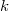$k$-chromatic Number of Paths

#### Abstract

A radio$k$-coloring of a graph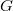$G$ is an assignment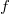$f$ of positive integers (colors) to the vertices of$G$ such that for any two vertices$u$ and$v$ of$G$, the difference between their colors is at least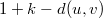$1+k-d(u,v)$. The span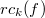$rc_k(f)$ of$f$ is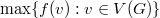$\max\{f(v):v\in V(G)\}$. The radio$k$-chromatic number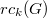$rc_k(G)$ of$G$ is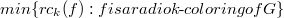$min\lbrace rc_k(f) : f { is a radio k\text{-}coloring of } G\rbrace$. In this paper, in an attempt to prove a conjecture on the radio$k$-chromatic number of path, we determine the radio$k$-chromatic number of paths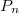$P_n$ for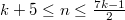$k+5\leq n\leq\frac{7k-1}{2}$ if$k$ is odd and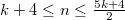$k+4\leq n\leq\frac{5k+4}{2}$ if$k$ is even.

DOI Code: 10.1285/i15900932v42n1p37